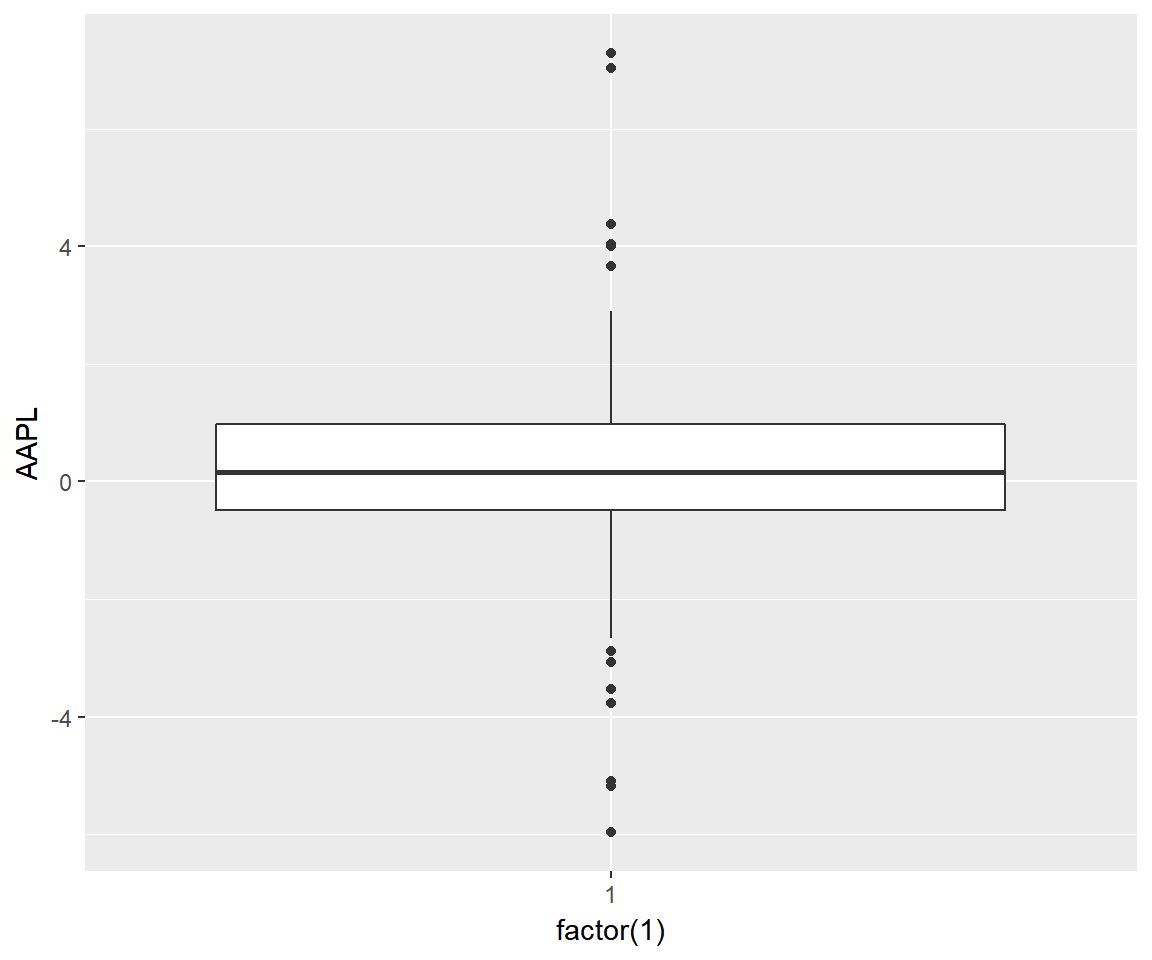## Agenda

• build box plots
• modify box
• color
• fill
• alpha
• line size
• line type
• modify outlier
• color
• shape
• size
• alpha

## Introduction

• the box plot is a standardized way of displaying the distribution of data
• box plots are useful for detecting outliers and for comparing distributions
• it shows the shape, central tendancy and variability of the data

## Structure

• the body of the boxplot consists of a “box” (hence, the name), which goes from the first quartile (Q1) to the third quartile (Q3)
• within the box, a vertical line is drawn at the Q2, the median of the data set
• two horizontal lines, called whiskers, extend from the front and back of the box
• the front whisker goes from Q1 to the smallest non-outlier in the data set, and the back whisker goes from Q3 to the largest non-outlier
• if the data set includes one or more outliers, they are plotted separately as points on the chart

## Libraries

``````library(ggplot2)

## Data

``daily_returns <- read_csv('https://raw.githubusercontent.com/rsquaredacademy/datasets/master/tickers.csv')``
``````## # A tibble: 250 x 5
##         AAPL       AMZN        FB       GOOG      MSFT
##        <dbl>      <dbl>     <dbl>      <dbl>     <dbl>
##  1  1.377845  24.169983  2.119995  22.409973  1.120701
##  2  2.834412   3.250000 -0.860001   5.989990  0.766800
##  3 -0.039360   9.910034  1.450005   6.750000  0.973240
##  4  0.108261   3.759949 -0.770004 -10.690002 -0.285091
##  5  1.643570  19.840027  4.750000   8.660034  0.501365
##  6  0.068894   5.330017 -0.299996  -0.929992  0.255596
##  7 -0.560975  -5.210022 -0.630005  -7.280030 -0.707809
##  8  0.551140   0.250000 -0.459999   0.690003  0.127796
##  9 -0.216522 -13.599975  0.030007   6.559997  0.078648
## 10 -0.108253  -4.250000  0.459999   2.600037  0.471878
## # ... with 240 more rows``````

## Univariate Box Plot

``````ggplot(daily_returns) +
geom_boxplot(aes(x = factor(1), y = AAPL))``````## Data

``tidy_returns <- read_csv('https://raw.githubusercontent.com/rsquaredacademy/datasets/master/tidy_tickers.csv')``
``````## # A tibble: 1,254 x 2
##    stock   returns
##    <chr>     <dbl>
##  1  AAPL  1.377845
##  2  AAPL  2.834412
##  3  AAPL -0.039360
##  4  AAPL  0.108261
##  5  AAPL  1.643570
##  6  AAPL  0.068894
##  7  AAPL -0.560975
##  8  AAPL  0.551140
##  9  AAPL -0.216522
## 10  AAPL -0.108253
## # ... with 1,244 more rows``````

## Box Plot

``````ggplot(tidy_returns) +
geom_boxplot(aes(x = factor(stock), y = returns))``````